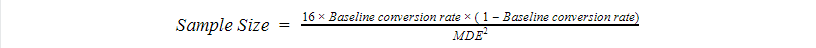Search
•Serhii Tolstoi

# How to calculate the sample size for the AB test?

You can use two options to calculate the sample size for the AB test:

1. use a sample size calculator

2. use math formula to calculate sample size for the AB test

1. Online Sample Size Calculator

I usually use Evan's sample size calculator. It's easy and fast to use.

You need just to specify some parameters as:

- Baseline conversion rate

- MDE (Minimum Detectable Effect)

- Power

- Significance LevelBelow you can find some terminology related to sample size calculation.

Baseline conversion rate - the expected rate of success in the control group. You can look at historical data on how this page has typically performed in the past. For example, a 20% conversion rate for the landing page.

Minimum Detectable Effect (MDE) - represents the relative minimum improvement over the baseline that you're willing to detect in an experiment. The smaller your MDE is, the larger the sample size required to reach statistical significance.

For example, with a 20% baseline conversion rate and a 5% MDE. Based on these values, your experiment will be able to detect 80% of the time when a variation's underlying conversion rate is actually 15% or 25% (20%, +/- 5%). If you try to detect differences smaller than 5%, your test is considered underpowered.

Power is a measure of how well you can distinguish the difference you are detecting from no difference at all. So running an underpowered test is the equivalent of not being able to strongly declare whether your variations are winning or losing.

A 5% significance level means that if you declare a winner in your AB test (reject the null hypothesis), then you have a 95% chance that you are correct in doing so. It also means that you have a significant result difference between the control and the variation with a 95% “confidence.” This threshold is, of course, an arbitrary one and one chooses it when making the design of an experiment.

Statistical significance answers the question, "How likely is it that my experiment results will say I have a winner when I actually don’t?” We usually consider a 95% statistical significance. Another way to say the same thing is that we will accept a 5% false-positive rate, where the result is not real (100% - 5% = 95%).

Whether or not the result can be called statistically significant depends on the p-value (known as alpha) we establish for significance before we begin the experiment. If the observed p-value is less than alpha, then the results are statistically significant.

A p-value is a probability of observing results at least as extreme as those measured when the null hypothesis is true.

2. Math formula to calculate sample size for the AB test:

When calculating the sample size, you will need to specify the significance level, power, minimum detectable effect (the desired relevant difference between the rates you would like to discover), and baseline conversion rate.Significance Level and Power are fixed values:Let's use 5% p-value and 80% power level, so we can simplify our formula to:Similarly, for the sample size for means we can use the next formula:Recommended articles: